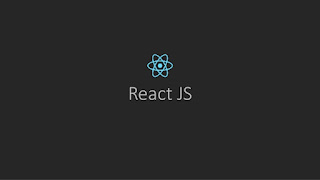### 7 New Features in PHP 7

PHP 7 is the first significant version update to PHP in several years. PHP 7 is much faster and, due to changes in the way errors are handled , more statble.It has several notable improvements and changes and  impressive benchmarks showing a 100% increase in performance.

Return type declarations
Scalar type hints
The space ship operator
Anonymous classes
The null coalesce operator
The Closure::call() method
Throwables

Return type declarations : Return type declarations has been introduced. Return type declaration specifies the type of value that a function should return. example of return type declartion are

```<?php
declare(strict_types = 1);
function returnIntValue(int \$value): int {
return \$value;
}
print(returnIntValue(5)); //output = 5
?>
```

Scalar type hints: Scalar type declarations come in two flavours: coercive (default) and strict. The following types for parameters can now be enforced (either coercively or strictly): strings (string), integers (int), floating-point numbers (float), and booleans (bool). They augment the other types introduced in PHP 5: class names, interfaces, array and callable.

```<?php
// Coercive mode
function sumOfInts(int ...\$ints)
{
return array_sum(\$ints);
}

var_dump(sumOfInts(2, '3', 4.1)); // int(9)
```

The space ship operator: What is the spaceship operator (<=>)? It is the newest operator in PHP and PHP’s very first “trirnary” operator. Previously, all other comparison operators in PHP returned either true or false. It is used to compare two expressions. It returns -1, 0 or 1 when first expression is respectively less than, equal to, or greater than second expression.

```<?php
//integer comparison
print( 1 <=> 1);print("<br/>");
print( 1 <=> 2);print("<br/>");
print( 2 <=> 1);print("<br/>");
print("<br/>");
//float comparison
print( 1.5 <=> 1.5);print("<br/>");
print( 1.5 <=> 2.5);print("<br/>");
print( 2.5 <=> 1.5);print("<br/>");
print("<br/>");
//string comparison
print( "a" <=> "a");print("<br/>");
print( "a" <=> "b");print("<br/>");
print( "b" <=> "a");print("<br/>");
?>
```

Anonymous : Those classes can now be defined using new class. Anonymous class can be used in place of a full class definition.

```<?php
/**
* Anonymous classes
*/
\$foo = new class {
public function foo() {
return "bar";
}
};
var_dump(\$foo,\$foo->foo());
```

The null coalesce operator : In PHP 5, we already have a ternary operator to check if else using shorthand.

```echo \$count ? \$count : 10; // outputs 10
```

It is a shorthand which allows you to skip the second element if it's the same as the first one:

```echo \$count ?: 10; // also outputs 10
```

In PHP 7 introduce new ?? operator which is indicating extreme confusion which is how I would usually use two question marks together instead allows us to chain together a string of values. Reading from left to right, the first value which exists and is not null is the value that will be returned.

```// \$a is not set
\$b = 16;

echo \$a ?? 2; // outputs 2
echo \$a ?? \$b ?? 7; // outputs 16
```

The Closure::call() method : Closures are anonymous functions that are declared inline and assigned to a variable. It is used as a callback for later execution.It is shorthand way to temporarily bind an object scope to a closure and invoke it.

```<?php
class A {
private \$x = 1;
}

// Define a closure Pre PHP 7 code
\$getValue = function() {
return \$this->x;
};

// Bind a clousure
\$value = \$getValue->bindTo(new A, 'A');

print(\$value()); // output 1
?>
```

Throwables : A major change in PHP 7 is the fact that errors are no longer raised the way they used to be raised. Errors now behave in a similar way as exceptions.

There are event different kinds of errors we can catch:
ArithmeticError
AssertionError
DivisionByZeroError
ParseError
TypeError

```<?php
/**
* Throwable interface
*/
//Error as Throwable
try {
sqdf();
} catch (Throwable \$t) {
echo "Throwable: ".\$t->getMessage().PHP_EOL;
}
//Exception as Throwable
try {
throw new Exception("Bla");
} catch (Throwable \$t) {
echo "Throwable: ".\$t->getMessage().PHP_EOL;
}
//Error
try {
sqdf();
} catch (Error \$e) {
echo "Error: ".\$e->getMessage().PHP_EOL;
} catch (Exception \$e) {
echo "Exception: ".\$e->getMessage().PHP_EOL;
}
//Exception
try {
throw new Exception("Bla");
} catch (Error \$e) {
echo "Error: ".\$e->getMessage().PHP_EOL;
} catch (Exception \$e) {
echo "Exception: ".\$e->getMessage().PHP_EOL;
}
//Type error
try {
function add(int \$a, int \$b):int {
return \$a + \$b;
}
} catch (TypeError \$t) {
echo "Type error: ".\$t->getMessage().PHP_EOL;
}
```